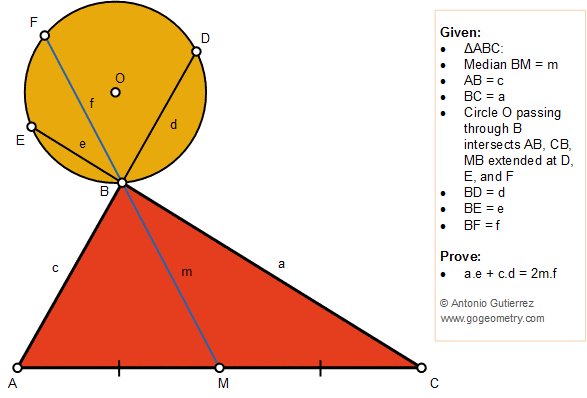# Geometry Problem 1383: Triangle, Median, Circle, Chord, Secant, Sum, Product

### Proposition

The figure below shows a triangle ABC with the median BM. A circle O passing through the vertex B intersects AB, CB, and MB at points D, E, and F, respectively. Prove that a.e + c.d = 2m.f